Volume 4, Issue 5, October 2016, Pages: 43-50

Definition of the Instantaneous Frequency of an Electroencephalogram Using the Hilbert Transform

Kharchenko Okcana

Physics and Technology Institute of Plasma Electronics and New Methods of Acceleration, National Science Center Kharkov Institute, Kharkiv, Ukraine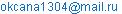Kharchenko Okcana. Definition of the Instantaneous Frequency of an Electroencephalogram Using the Hilbert Transform. Advances in Bioscience and Bioengineering. Vol. 4, No. 5, 2016, pp. 43-50. doi: 10.11648/j.abb.20160405.11

Received: July 11, 2016; Accepted: August 5, 2016; Published: August 31, 2016

Abstract: Application of methods of signal processing used in radioengineering for electroencephalograms (EEG) provides to increase the information content of the analysis and medical diagnostic quality. In this paper the method of EEG analysis based on the Hilbert transform are considered. Comparing results analysis with the Fourier transform (FT) and wavelet transform (WT) is carried out. Using the Hilbert transform for calculations, the analysis data on the informative parameters of EEG of healthy persons and those of epileptic patients were obtained. The calculated time dependences of the total phase and instantaneous frequency are presented on the diagrams. It is shown that application of the Hilbert transform provides an evident and simple interpretation of EEG diagnostics results. The phase-frequency method of EEG analysis gives an opportunity to track the dynamics of EEG change, to numerically characterize the duration and variation of the basic physiological rhythms, and, also, to observe the frequency change in time within the limits of each rhythm.

Keywords: Electroencephalogram, Hilbert Transform, Instantaneous Frequency, EEG Rhythms

Contents

1. Introduction

Since its discovery, EEG has been useful tool in understanding of brain functioning at all and diagnosing neurophysiological disorders. Usually clinicians and scientists investigated EEG patterns by visual inspection or by limited quantitative analysis of rhythms in the waveforms that were printed on EEG chart papers, that required a high qualification of analyst. Signal processing based on radioengineering methods resulted in an incentive to use computer technology, automate detection and analysis, and use more objective quantitative approaches. The use of computers in EEG enables real-time denoising, automatic rhythmic analysis, and more complicated quantifications.

Nowadays the digital methods of EEG analysis (in main, spectral and correlation analysis) are finding ever increasing application. An advantage of these methods consists in the elimination of subjectivity, being inevitable for a visual analysis. EEG is a nonstationary process, but in the numerical methods this fact, as a rule, is not taken into account [1,2]. The wavelet transform is used to analyze non-stationary processes . But this method is not yet used extensively in the diagnostics by specialists because of complexity in interpretations of results.

Spectral and correlation analysis allow one to determine the domination of certain frequency components in the given section of EEG and, using these data, to reveal, in particular, a zone of pathologic center. However, they do not give an opportunity to track and to characterize numerically in detail the time change of the EEG structure, variation and duration of rhythms, that is of current importance taking into account the EEG nonstationarity.

2. Research Methods and Materials

The study involved 40 subjects were men aged 25 to 50 years. Of these, 15 patients were suffering from epilepsy. The EEG recording was carried out with the help of electroencephalograph EEG 16S "Medicor" (made in Hungary) with the positioning of the electrodes according to the international 10-20 system.

The wavelet transform has become a useful computational tool for a variety of signal. The wavelet transform is designed for signal analysis. For example, to study EEG or other biomedical signals, to determine how the frequency content of a signal evolves over time. The wavelet transform is similar to the Fourier transform (or much more to the windowed Fourier transform) with a completely different merit function. The main difference is this: Fourier transform decomposes the signal into sines and cosines, i.e. the functions localized in Fourier space; in contrary the wavelet transform uses functions that are localized in both the real and Fourier space. Generally, the wavelet transform can be expressed by the following equation: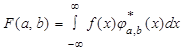,

where the * is the complex conjugate symbol and function ψ is some function. This function can be chosen arbitrarily provided that it obeys certain rules.

The Hilbert transform is based on the representation of a random process p(t) in the form of p(t)=A(t)cosφ(t) [4,5]. Thus, the random process p(t) is considered as a harmonic fluctuation modulated in amplitude and in phase by the stochastic functions p(t) and φ(t). The calculation of these functions allows one to observe the change in amplitude and in phase of EEG with time, and, also, to investigate the time change of instantaneous and average frequencies of fluctuations, if to approach to the concept of frequency as a derivative of the total phase of the oscillation process. Such a method of analysis allows to characterize numerically the duration and frequency of the change of separate rhythms.

The opportunity of representing the random process as a harmonic fluctuation randomly modulated in amplitude and in phase, has anenough deep theoretical justification [4,5]. By writing the random process p(t) in the form of

p(t)=A(t)cosφ(t),(1)

it is possible to establish the relation between A(t), p(t) and φ(t) in terms of the Hilbert transform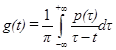.

The function g(t) is a Hilbert conjugate process with respect to p(t).

The envelope A(t) and the phase φ(t) in (1) are determined as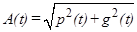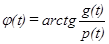(2)

A representation of random process in the form of (1) is easy interpreted for the narrow-band processes. In this case the concept of the envelope acquires a fair obviousness. Besides, it is possible to determine the instantaneous frequency of the process as follows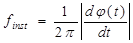(3)

EEG is a broad-band process and, in this case, the concept of the envelope has not the precise physical sense [4,5]. At the same time, the analysis of the function φ(t) allows obtaining some additional information on the stability of frequency characteristics, duration of stationary, and on the character of transient mode sin the oscillation process p(t)=A(t)cosφ(t) [6,7].

Let us demonstrate that for the broad–band processes the introduction of the concept of the instantaneous frequency enables to characterize the duration and the rate of change of characteristic fluctuations (rhythms in the EEG case) in the process under study.

3. Results and Discussion

Consider, for example, EEG of a healthy adult person (Fig. 1). The subject is in a state of quiet wakefulness. In this condition α-rhythm is usually dominated. In practice α-rhythm is characterized by a frequency in the range of 8 to 13 Hz, amplitude up to 100 µv and recorded at 85÷95% of healthy adults examined. α-rhythm is best expressed in the occipital departments and in the direction anteriorly its amplitude gradually decreases. Healthy people are characterized by a relatively narrow frequency range of α-rhythm. Amplitude of α-rhythm changes significantly in time. Fairly regularly observed spontaneous changes in the amplitude, the so-called "spindles", which is expressed in an alternating increase and decrease in amplitude. In Fig. 1 it is possible to observe these spindles.Figure 1. EEG realization of a healthy adult.

Define the FT of the EEG realization of a healthy adult (Fig. 2). Intervals for different rhythms plotted in Fig. 2. In this figure is possible to observe a high level of α-rhythm that is natural in a state of quiet wakefulness . Other rhythms are weak. Note that you can not see it slow oscillation between 4 and 5 seconds.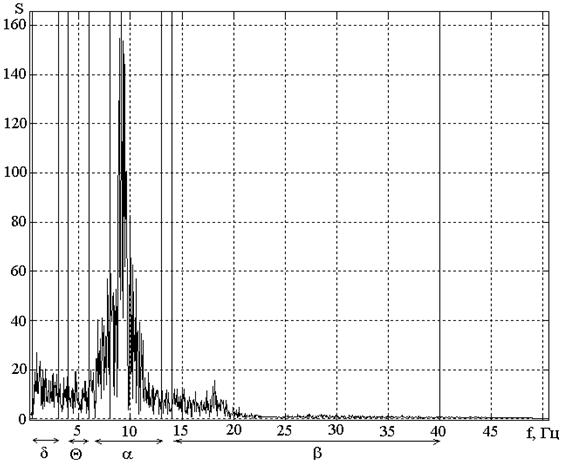Figure 2. Fourier transform ofthe EEG realization in Fig. 1.

Fig. 3 shows Wavelet transform to the EEG implementation shown in Fig. 1 using Morlet wavelets. Obviously that alpha-rhythm dominates in this signal. Besides virtually invisible oscillations that take place between 4 and 5 second are revealed quite weakly.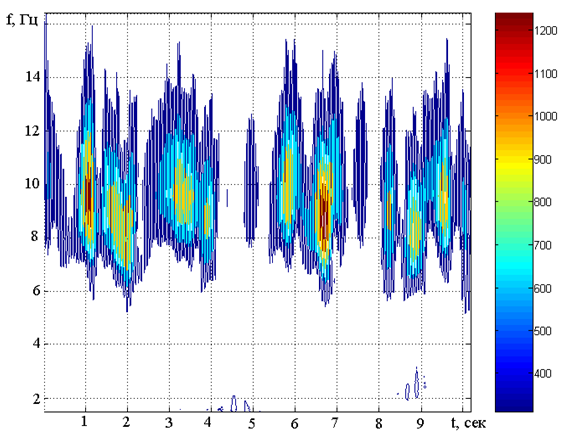Figure 3. Wavelet transform ofthe EEG realization in Fig. 1.

The time dependence of the EEG total phase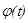may be determined by expression (2). The result of calculation is presented in Fig. 4. This graph is almost a straight line, indicating on the constancy of the increment of phase, and hence small frequency deviation.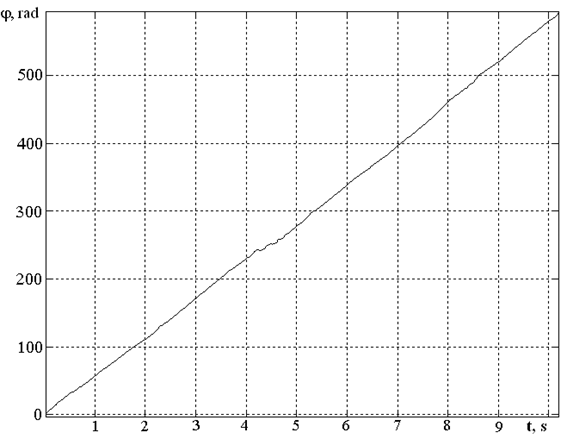Figure 4. A phase curve corresponding to the EEG realization in Fig. 1.

Further, by writing a total phase as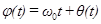, where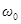is the average frequency of the process, we obtain: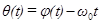. The average frequency is determined by the regression line (Fig. 5) and shows that phase dependence is close to straight line. In addition, this graph can be divided into straight line segments. Each segment can be considered as stationary phase of the EEG.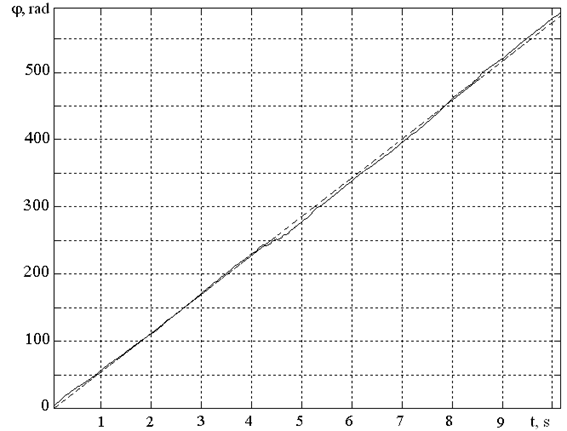Figure 5. A phase curve (solid line) and a regression line (dotted line) corresponding to the EEG realization in Fig. 1.

There are represented (Fig. 6) the results of instantaneous frequencies of the EEG process calculated by formula (3).

It is seen that the frequency of changes, mostly within the α-rhythm range. Consider the point of fall of frequency (in the interval from 4 to 5 s). In Fig. 1 visually shows the slow oscillation in the interval from 4 to 5 seconds. It has low amplitude and are not detected using Fourier transform and Wavelet transform (Fig. 2, 3). But with the help of the Hilbert transform we can detect this fluctuation, because at this point the α-rhythm has low power, that can be seen from Fig. 1. Fig. 7 shows the relatively low deviation of instant frequency of a signal from average value. We can see that phase of EEG is characterized by relative stability and insignificant deviations from the regression line.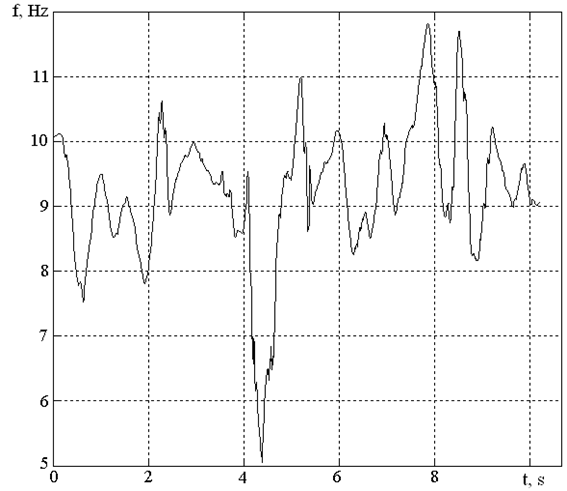Figure 6. An instantaneous frequency-time diagram corresponding to the EEG realization in Fig. 1.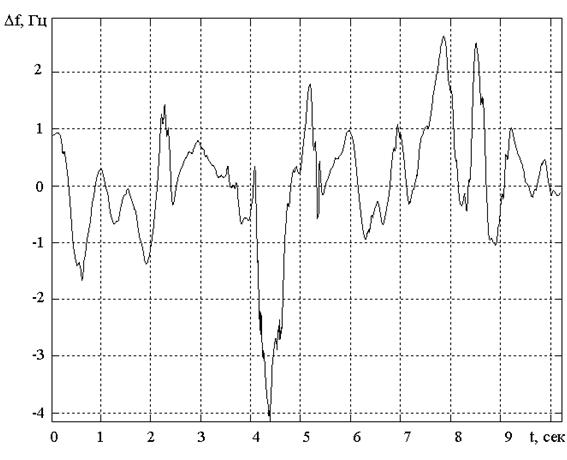Figure 7. Frequency fluctuation vs. time, corresponding to realization EEG in Fig. 1.

As numerical estimations of EEG in the medical diagnostics one uses the index of rhythm  representing the relative duration of a rhythm in the given epoch of the EEG realization, the average frequency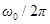and the dispersion of frequency of each rhythm 

Under the index of rhythm understand the percentage of time the activity rhythm recorded on the EEG. To determine the index of rhythm determine the length of curve segments, which recorded the rhythm and expressed in percentage. The EEG of different individuals alpha-index ranges from 0 to 100. Normally it is 75-95%, and below 50% is regarded as pathology.

The calculation results on the specified parameters for realization of Fig. 1 are given in Table 1.

Table 1. Information Parameters of EEGof a Healthy Adult.

 Rhythm Index of rhythm,% Average value of frequency, Hz Dispersion of frequency, Hz2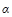91.42 9.44 0.57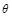1.33 5.44 0.06

The obtained results show that in the EEG prevailing signal realizations is the component of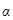-rhythm (the index of rhythm exceeds 90%). We should also note almost full absence of low-frequency components.

EEG realization of the epileptic patient are presented in Fig. 8. This signal differs from the EEG of a healthy adult even visually.

In Fig. 9 shows FT of this realization. It contains strong frequency components in the ranges of θ- and δ-rhythms and almost no contains α-rhythm.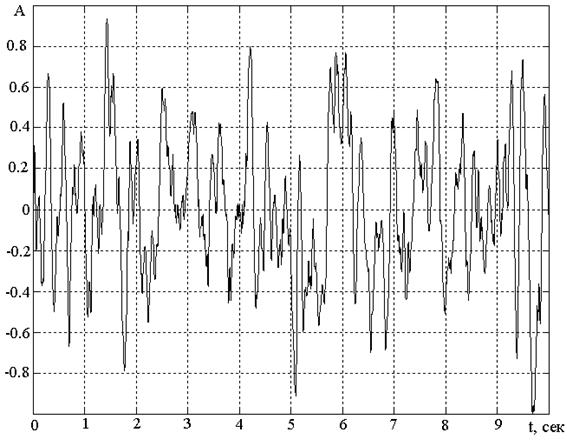Figure 8. EEG realization of the epileptic patient.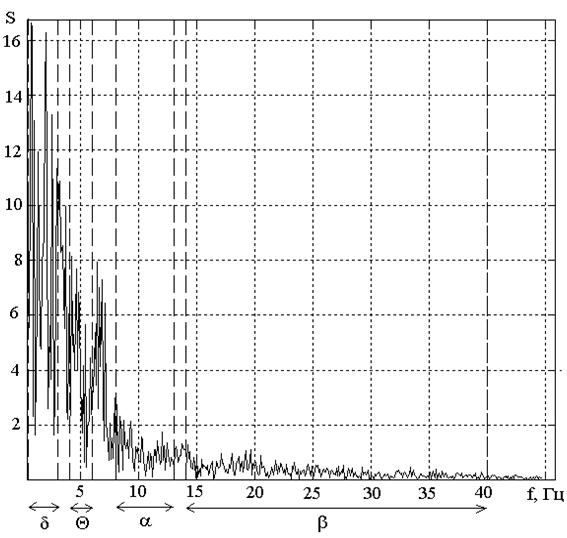Figure 9. Fourier transform ofthe EEG realization in Fig. 8.

In Fig. 10 shows Wavelet transform implementation shown in Fig. 8 using Morlet wavelets. It is seen that the main power of this EEG is concentrated at low frequencies.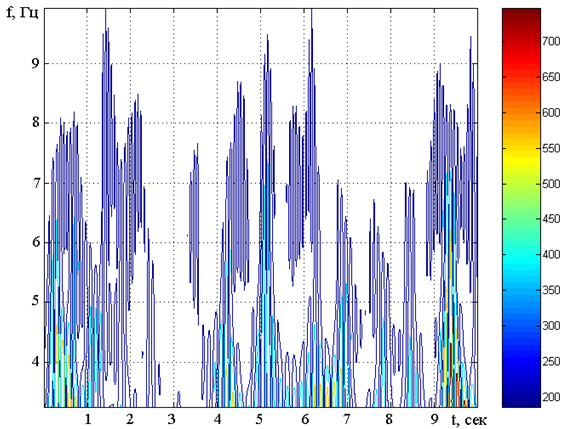Figure 10. Wavelet transform ofthe EEG realization in Fig. 8.

Fig. 11 illustrate the total phase time dependence of the EEG for epileptic patient. Comparing Fig. 11 and Fig. 4 we see, that phase curve of a healthy adultis significantly uniform while for epileptic patient it differs by the sharp breaks. Besides, the phase curve analysis allows to allocate the EEG stationary segment.

Regression line corresponding to the EEG realization of the epileptic patient is shown in Fig. 12. Here the average frequency is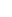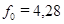Hz that is significantly low then the average frequency measured for the healthy adult.

The diagram for instantaneous frequency in the case of realization of the epileptic patient’s EEG is shown in Fig. 13.

Pathological manifestations on EEG are the emergence of slow rhythms so called θ- and δ-rhythms. The lower the frequency and higher the amplitude of rhythm, the more pronounced the pathological process. The θ-rhythm is characterized by a frequency of 4÷6 Hz, amplitude exceeds 40 μV, reaching in some pathological conditions 300 mV or more. The δ-rhythm is characterized by a frequency of 0.5÷3 Hz, the amplitude is the same as θ-rhythm. On the EEG of waking adult person can meet pieces of θ- and δ-rhythms of short duration and with an amplitude not exceeding the amplitude of the α-rhythm. In this case we speak about a certain decrease of the level of functional activity of the brain. In Fig. 13 are observed in almost all the rhythms that evidence pathology. In addition, the frequency changes drastically and in widely range.

The frequency instability is obvious in comparison with the realization of the healthy adults EEG. Frequency deviation has a high level as well (Fig. 13).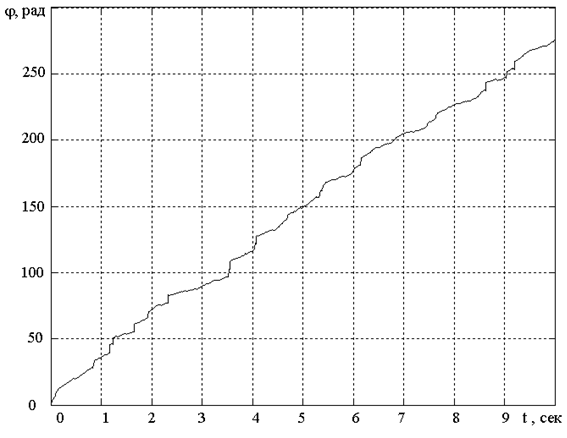Figure 11. The phase curve corresponding to the EEG realization in Fig. 8.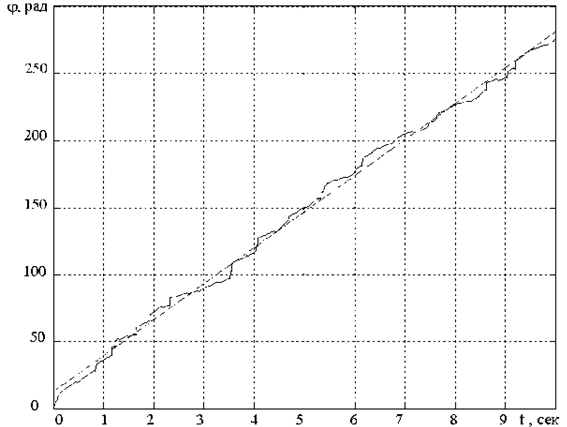Figure 12. A phase curve (solid line) and a regression line (dotted line) corresponding to the EEG realization in Fig. 8.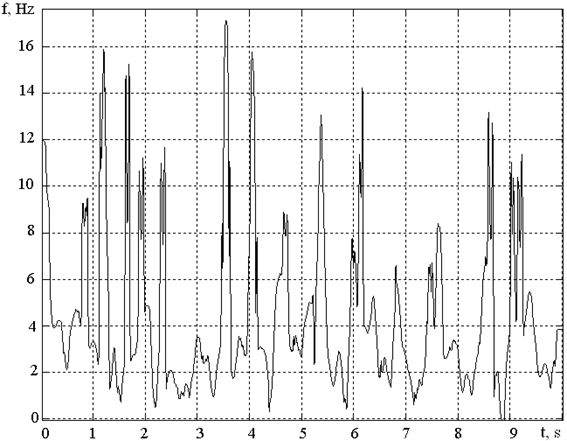Figure 13. An instantaneous frequency–time diagram, corresponding to the EEG realization in Fig. 8.

Fig. 13 shows the high deviation of instant frequency of a signal from average value.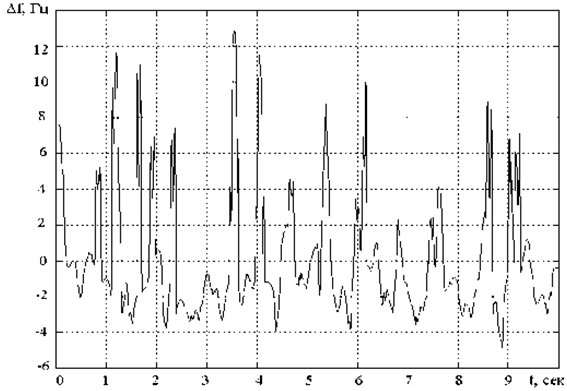Figure 14. Frequency fluctuation vs. time, corresponding to realization EEG in Fig. 8.

The calculation results on the index of rhythm, average value and frequency dispersion of each rhythm are given in Table 2.

Table 2. Information Parameters of EEGof an Epileptic Patient.

 Rhythm Index ofrhythm,% Average value of frequency, Hz Dispersion of frequency, Hz212.03 9.99 1.9715.98 4.79 0.29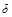44.14 1.98 1.42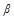2.11 15.47 0.99

In the realization of the epileptic patient’s EEG the low-frequency component is appreciably increased (the index of-rhythm exceeds 44%) that evidences on a probable pathology.

Investigations were carried out on the samples obtained from 40 persons . The comparison of instantaneous frequency-time diagrams has shown that the frequency deviations from the average value are much higher for epileptic patients than for healthy persons.

4. Conclusion

The phase-frequency method of EEG analysis gives an opportunity to track the dynamics of EEG change, to haracterize numerically the duration and variation of the basic physiological rhythms, and, also, to observe the frequency change in time within the limits of each rhythm.

Besides, the phase-frequency method of EEG analysis enables to reveal changes in the instantaneous frequency and fluctuation phase provoked by episodicirritations. With the help of this method it is possible to record changes in EEG in the case of pathology, in particular, occurrence of an epileptogenic activity.

Now EEG is considered as a series the stationary segment. The segmentation process is an important part of EEG signal processing. The aim of the segmentation is to separate quasi stationary parts of the recorded EEG signals to the segments, in which the selected properties are stationary [9,10]. A phase curve may be used as a new segmentation method of EEG.

Simplicity and visual evidence of this method, as well as, an opportunity of its hardware or software realization in real time are important for mass examinations and initial diagnostics.

It should be noted also a disadvantage of this method, namely, the ability to separate only a single high-amplitude component from the continuum of frequencies which take place in the short part of the analyzed signal.

References

1. L. P. Zenkov. Clinical electroencephalography (with elements of epileptology) / Doctors’ manual. – M., Medpressinform, 2004-368 p. (in Russian).
2. Campbell J. et al. On the sufficiency of autocorrelation functions as EEG descriptors // IEEE Trans. Bio-Med. Eng. – 1967. – V. BME-14. – P. 49-52.
3. Zhang Xizheng, Yin Ling, Wang Weixiong. Wavelet time-frequency Analysis of Electro-encephalogram (EEG) processing // (IJACSA) International Journal of Advanced Computer Science and Applications, Vol. 1, No. 5, November 2010. –P. 1-5.
4. N. D. Devyatkov, O. M. Grindel, I. F. Kharchenko et al. Investigations on the instability of time characteristics of human EEG by the method of phase-frequency analysis // Vestnik Akademii Meditsinskikh Nauk SSSR.-M.: Meditsina.-1973.-45 p. (in Russian).
5. Yu. I. VoloshchukSignals and processes in radio engineering.- Kharkov: CMIT, 2003, - 444 p. (in Ukrainian).
6. V. I. Tikhonov Statistical radio engineering.-M.: Sovetskoye Radio, 1966.-678 p. (in Russian).
7. I. S. Gonorovsky. Radiotechnical circuits and signals.- M.: Sovetskoye Radio, 1966. Vol. 1. – 439 p. (in Russian).
8. O. I. Kharchenko. Methods of frequency- time analysis in the systems of electroencephalogram-type random signal processing. Ph.D. dissertation on technical sciences.-Kharkov: Kharkov National University of Radio Electronics.- 2007.-253 p. (in Russian).
9. Sara Marianie. a. EEG segmentation for improving automatic CAP detection // Clinical Neurophysiology Volume 124, Issue 9, September 2013.- P 1815–1823.
10. Tsuyoshi Inouye, SeigoToi, Yuko Matsumoto A new segmentation method of electroencephalograms by use of Akaike's information criterion // Cognitive Brain Research, Volume 3, Issue 1, December 1995. – P. 33–40.

 Contents 1. 2. 3. 4.
Article ToolsAbstractPDF(1421K)